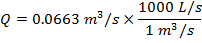# Flow Rate Formula

Flow Rate Formula

The flow rate of a liquid is a measure of the volume of liquid that moves in a certain amount of time. The flow rate depends on the area of the pipe or channel that the liquid is moving through, and the velocity of the liquid. If the liquid is flowing through a pipe, the area is A = πr2, where r is the radius of the pipe. For a rectangle, the area is A = wh where w is the width, and h is the height. The flow rate can be measured in meters cubed per second (m3/s), or in liters per second (L/s). Liters are more common for measures of liquid volume, and 1 m3/s = 1000 L/s.

fluid flow rate = area of the pipe or channel×velocity of the liquid

Q = Av

Q = liquid flow rate (m3/s or L/s)

A = area of the pipe or channel (m2)

v = velocity of the liquid (m/s)

Flow Rate Formula Questions:

1) Water is flowing through a circular pipe that has a radius of 0.0800 m. The velocity of the water is 3.30 m/s. What is the flow rate of the water in liters per second (L/s)?

Answer: The flow rate depends on the area of the circular pipe:

A = πr2

A = π(0.0800 m)2

A = π(0.00640 m2)

A = 0.0201 m2

The area of the pipe is 0.0201 m2. The flow rate can be found in m3/s using the formula:

Q = Av

Q = (0.0201 m2)(3.30 m/s)

Q = 0.0663 m3/s

The flow rate can be converted to liters per second using: 1 m3/s = 1000 L/s.Q = 66.3 L/s

The flow rate of the water through the circular pipe is 66.3 L/s.

2) Water is flowing down an open rectangular chute. The chute is 1.20 m wide, and the depth of water flowing in it is 0.200 m. The velocity of the water is through a circular pipe that has a radius of 0.0800 m. The velocity of the water is 5.00 m/s. What is the flow rate of the water through the chute in liters per second (L/s)?

Answer: The flow rate depends on the area of the chute the water is flowing through:

A = wh

A = (1.20 m)(0.200 m)

A = 0.240 m2

The area of the water as it flows through the chute is 0.240 m2. The flow rate can be found in m3/s using the formula:

Q = Av

Q = (0.240 m2)(5.00 m/s)

Q = 1.20 m3/s

The flow rate can be converted to liters per second using: 1 m3/s = 1000 L/s.Q = 1200 L/s

The flow rate of the water in the chute is 1200 L/s.